﻿ 形变观测序列变振幅年周期探测方法研究文章快速检索 高级检索
 大地测量与地球动力学2020, Vol. 40Issue (1): 7-10, 86  DOI: 10.14075/j.jgg.2020.01.002### 引用本文WANG Wei, LI Hongyu, YE Biwen, et al. Research on the Method for Annual Period Detection of Variable Amplitude for the Time Series of Deformation[J]. Journal of Geodesy and Geodynamics, 2020, 40(1): 7-10, 86.### Foundation support

The Earthquake Tracking Task of CEA, No. 2018010205; Director Fund of Jiangsu Earthquake Agency, No.17410.

### 文章历史

1. 江苏省地震局，南京市卫岗3号，210014

1 年变探测方法 1.1 最小二乘拟合

 \begin{aligned} y(t)=& y_{0}+v t_{i}+a \sin \left(2 \pi f t_{i}\right)+\\ & b \cos \left(2 \pi f t_{i}\right)+\varepsilon\left(t_{i}\right) \end{aligned} (1)

 $\boldsymbol{y}=\boldsymbol{A x}+\boldsymbol{e}, D(\boldsymbol{y})=\sigma_{0}^{2} \boldsymbol{P}^{-1}$ (2)

 $\left\{\begin{array}{ll} {\boldsymbol{A}=\left[\begin{array}{cccc} {1} & {t_{1}} & {\sin \left(2 \pi f t_{1}\right)} & {\cos \left(2 \pi f t_{1}\right)} \\ {1} & {t_{2}} & {\sin \left(2 \pi f t_{2}\right)} & {\cos \left(2 \pi f t_{2}\right)} \\ {\vdots} & {\vdots} & {\vdots} & {\vdots} \\ {1} & {t_{n}} & {\sin \left(2 \pi f t_{n}\right)} & {\cos \left(2 \pi f t_{n}\right)} \end{array}\right]} \\ {\boldsymbol{x}=\left[\begin{array}{cccc} {y_{0}} & {v} & {a} & {b} \end{array}\right]^{{\rm T}}} \end{array}\right.$ (3)
1.2 基于F检验的分段最小二乘拟合

 $\left\{ \begin{array}{l} {\mathit{\boldsymbol{A}}_k} = \left[ {\begin{array}{*{20}{c}} 1&{{t_1}}&{\sin (2{\rm{ \mathsf{ π} }}f{t_1})}&{\cos (2{\rm{ \mathsf{ π} }}f{t_1})}&0&0\\ 1&{{t_2}}&{\sin (2{\rm{ \mathsf{ π} }}f{t_2})}&{\cos (2{\rm{ \mathsf{ π} }}f{t_2})}&0&0\\ \vdots & \vdots & \vdots & \vdots & \vdots & \vdots \\ 1&{{t_k}}&{\sin (2{\rm{ \mathsf{ π} }}f{t_k})}&{\cos (2{\rm{ \mathsf{ π} }}f{t_k})}&0&0\\ 1&{{t_{k + 1}}}&0&0&{\sin (2{\rm{ \mathsf{ π} }}f{t_{k + 1}})}&{\cos (2{\rm{ \mathsf{ π} }}f{t_{k + 1}})}\\ \vdots & \vdots & \vdots & \vdots & \vdots & \vdots \\ 1&{{t_n}}&0&0&{\sin (2{\rm{ \mathsf{ π} }}f{t_n})}&{\cos (2{\rm{ \mathsf{ π} }}f{t_n})} \end{array}} \right]\\ {\mathit{\boldsymbol{x}}_k} = {\left[ {\begin{array}{*{20}{c}} {{y_0}}&v&{{a_1}}&{{b_1}}&{{a_2}}&{{b_2}} \end{array}} \right]^{\rm{T}}} \end{array} \right.$ (4)

 ${\mathit{\boldsymbol{\hat \sigma }}} _k^2 = \frac{{{{\left( {{\mathit{\boldsymbol{y}}} - {{\mathit{\boldsymbol{A}}}_k}{{{\mathit{\boldsymbol{\hat x}}}}_k}} \right)}^{\rm{T}}}{\mathit{\boldsymbol{P}}}\left( {{\mathit{\boldsymbol{y}}} - {{\mathit{\boldsymbol{A}}}_k}{{{\mathit{\boldsymbol{\hat x}}}}_k}} \right)}}{{n - {m_k}}}$ (5)

 $F = \frac{{{\mathit{\boldsymbol{\hat \sigma }}} _0^2}}{{{\mathit{\boldsymbol{\hat \sigma }}} _p^2}}\sim{F_\alpha }\left( {n - m, n - {m_p}} \right)$ (6)

1.3 滑动傅里叶法

 $\begin{array}{c} {x_n} = {a_n}\cos \frac{{2{\rm{ \mathsf{ π} }}\left( {j - n + T} \right)}}{T} + \\ {b_n}\sin \frac{{2{\rm{ \mathsf{ π} }}\left( {j - n + T} \right)}}{T}\\ n = T, T + 1, \cdots , N;j = \\ n - T + 1, n - T + 2, \cdots , n \end{array}$ (7)

 ${a_n} = \frac{2}{T}\sum\limits_{j = n - T + 1}^n {{y_i}\cos \frac{{2{\rm{ \mathsf{ π} }}\left( {j - n + T} \right)}}{T}}$ (8a)
 ${b_n} = \frac{2}{T}\sum\limits_{j = n - T + 1}^n {{y_i}\sin \frac{{2{\rm{ \mathsf{ π} }}\left( {j - n + T} \right)}}{T}}$ (8b)

2 数据分析 2.1 原始数据预处理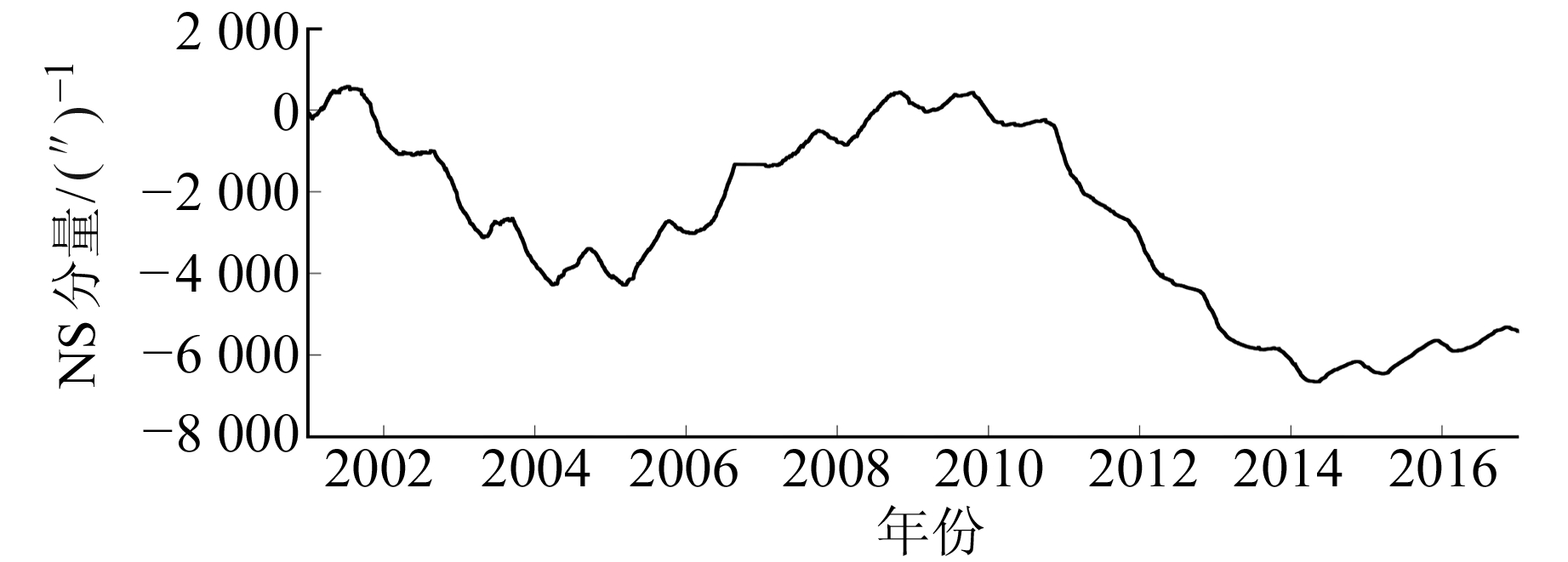图 1 库尔勒水平摆倾斜仪NS分量日值曲线 Fig. 1 The curve of daily observation of Korla horizontal pendulum tiltmeter

2.2 变振幅年周期信号提取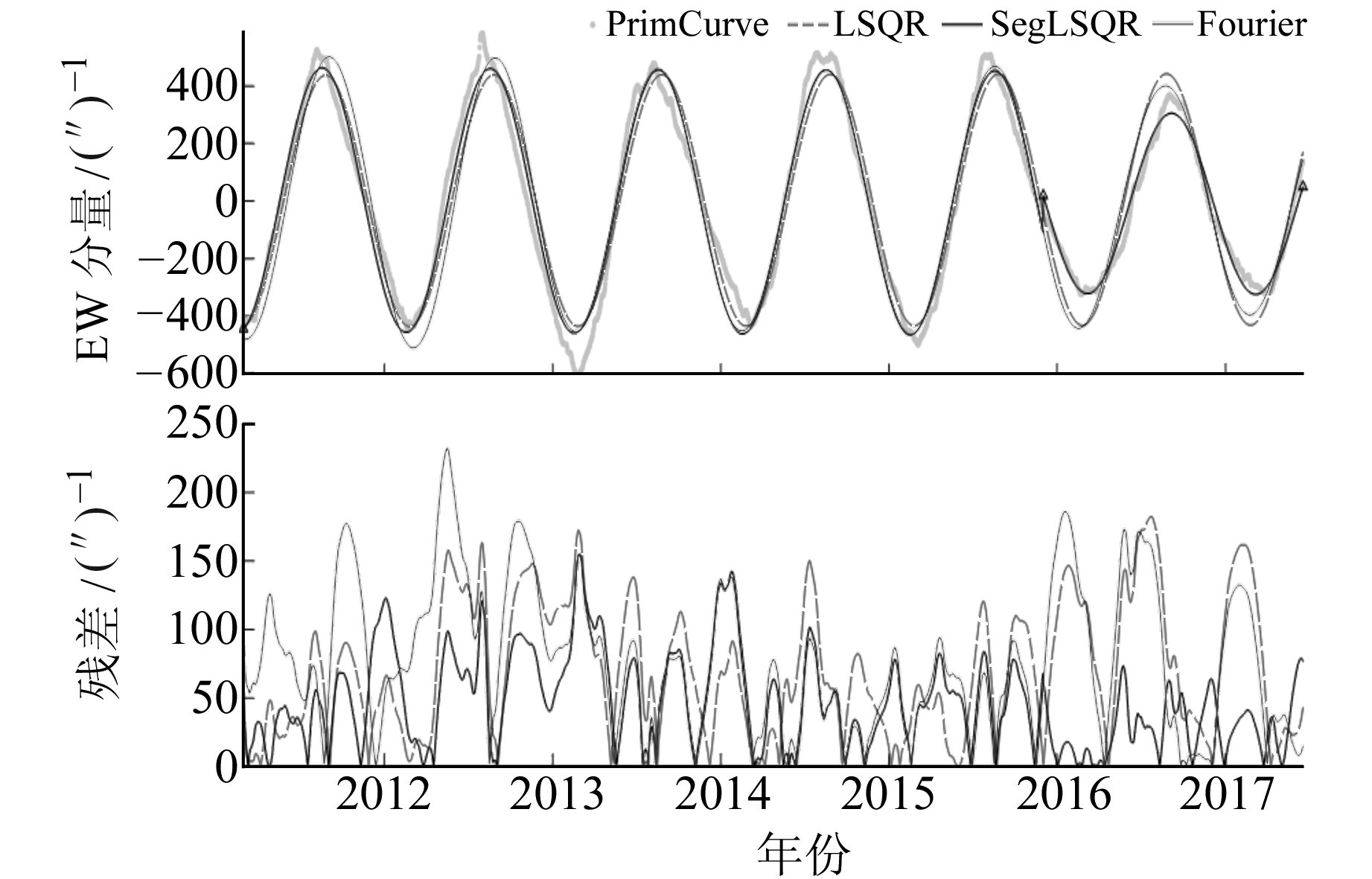图 2 温泉水平摆倾斜仪EW分量拟合和残差曲线 Fig. 2 The fitting curves and theresiduals of Wenquan horizontal pendulum tiltmeter(EW)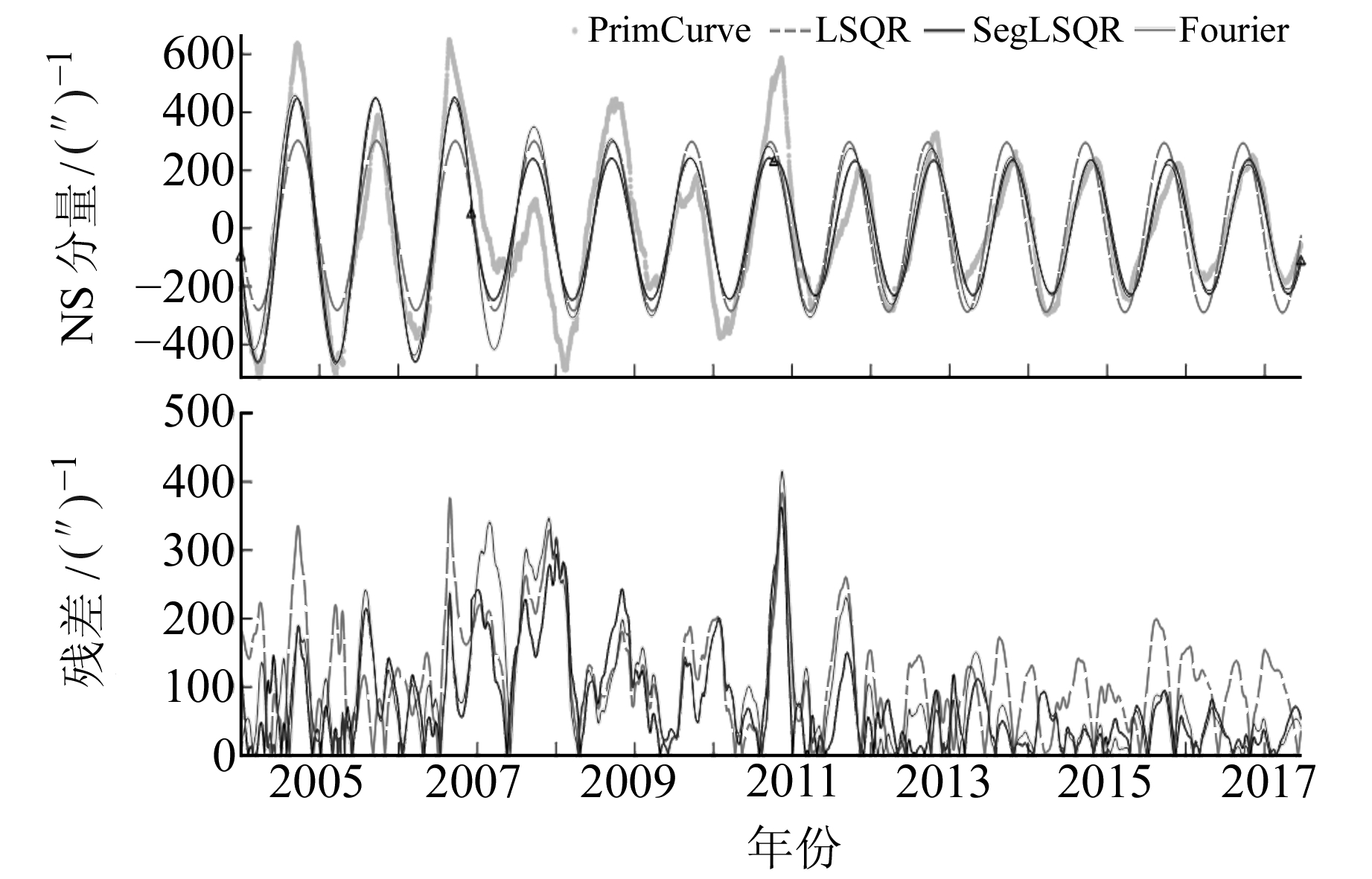图 3 库尔勒水平摆倾斜仪NS分量拟合和残差曲线 Fig. 3 The fitting curves and theresiduals of Korla horizontal pendulum tiltmeter(NS)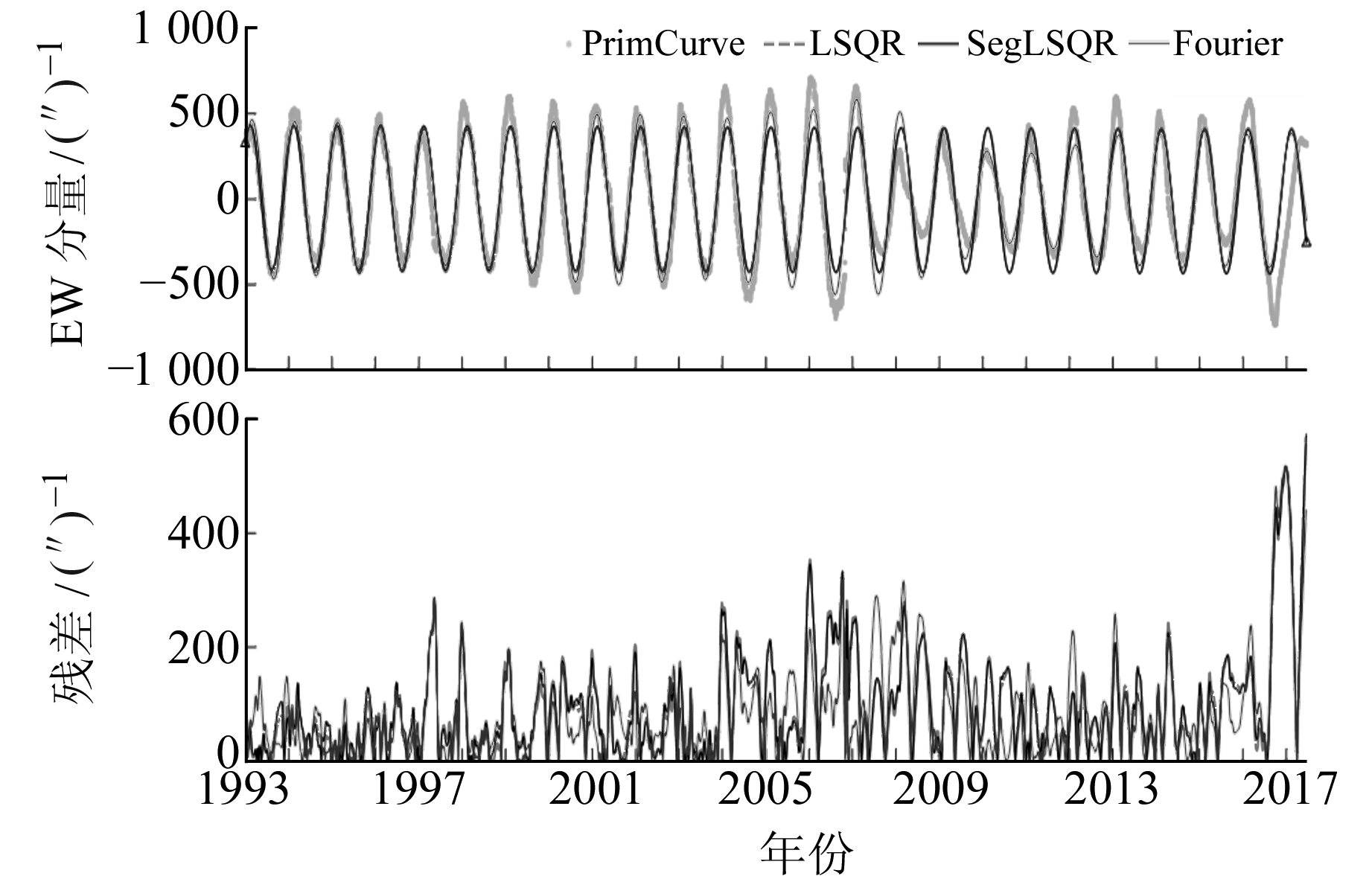图 4 精河水平摆倾斜仪EW分量拟合和残差曲线 Fig. 4 The fitting curves and theresiduals of Jinghe horizontal pendulum tiltmeter(EW)表 1 3种方法拟合结果标准差 Tab. 1 The standard deviations of the three methods
2.3 利用谱分析判断年周期信号提取效果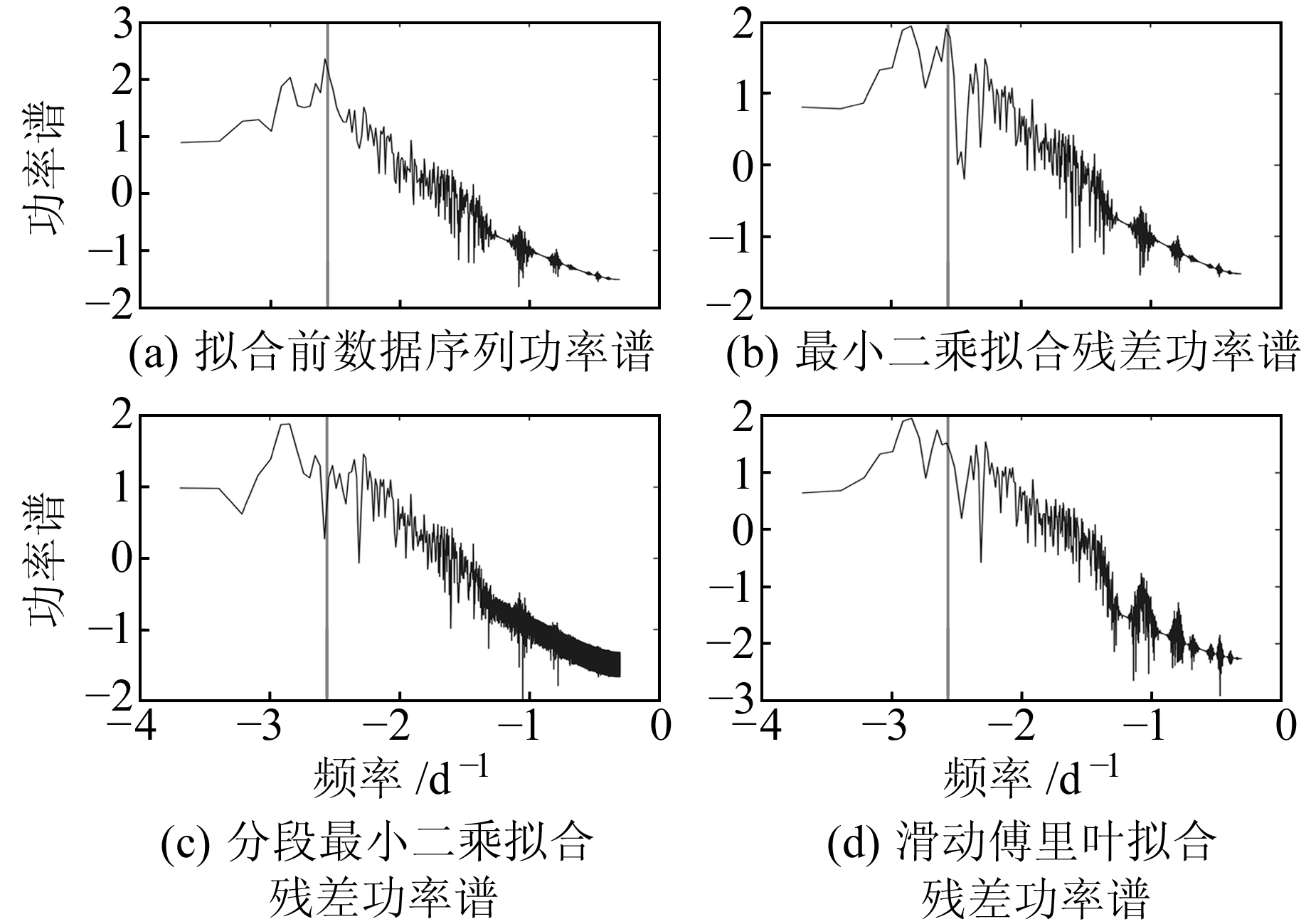图 5 功率谱分析 Fig. 5 Spectrum analysis
3 结语

1) 最小二乘法受模型限制，仅能拟合出固定振幅、固定相位的年周期信号，对年周期变化比较规律的曲线拟合程度高、残差小，但对变振幅年周期的拟合效果较差。

2) 基于F检验的分段最小二乘法，在最小二乘基础上增加分段点的自动搜索，可自适应振幅改变的情况。该方法既继承了最小二乘法的优点，又顾及到观测数据年周期形态的复杂多样性，从3个测项年周期拟合残差的标准差也能看出，该方法拟合效果最好。对于个别相位改变的年周期，分段最小二乘法也能较好地拟合出来，但分段点不连续，存在分段台阶或周期不连续现象。

3) 滑动傅里叶法在数据较长、振幅改变的情况下拟合效果最优。该方法可通过前3 a数据调整拟合参数，年周期振幅缓慢持续改变的拟合结果明显优于年周期振幅突变的拟合结果。与最小二乘法一样，滑动傅里叶法以365 d为拟合周期，且未考虑相位参数，因此对于相位改变的拟合结果与原始数据序列存在相位差。

  Dong D, Fang P, Bock Y, et al. Anatomy of Apparent Seasonal Variations from GPS-Derived Site Position Time Series[J]. Journal of Geophysical Research: Solid Earth, 2002, 107(B4) (0)  姜卫平, 夏传义, 李昭, 等. 环境负载对区域GPS基准站时间序列的影响分析[J]. 测绘学报, 2014, 43(12): 1217-1223 (Jiang Weiping, Xia Chuanyi, Li Zhao, et al. Analysis of Environmental Loading Effects on Regional GPS Coordinate Time Series[J]. Acta Geodaetica et Cartographica Sinica, 2014, 43(12): 1217-1223) (0)  Davis J L, Wernicke B P, Tamisiea M E. On Seasonal Signals in Geodetic Time Series[J]. Journal of Geophysical Research: Solid Earth, 2012, 117(B1) (0)  黄立人. GPS基准站坐标分量时间序列的噪声特征分析[J]. 大地测量与地球动力学, 2006, 26(2): 31-33 (Huang Liren. Noise Properties in Time Series of Coordinate Component at GPS Fiducial Stations[J]. Journal of Geodesy and Geodynamics, 2006, 26(2): 31-33) (0)  江在森, 张希, 祝意青, 等. 昆仑山口西MS8.1级地震前区域构造变形背景[J]. 中国科学D辑, 2003, 33(增1): 163-172 (Jiang Zaisen, Zhang Xi, Zhu Yiqing, et al. Regional Tectonic Deformation Background before the MS8.1 Earthquake in the West of Kunlun Pass[J]. Science in China Series D, 2003, 33(S1): 163-172) (0)  王解先, 连丽珍, 沈云中. 奇异谱分析在GPS站坐标监测序列分析中的应用[J]. 同济大学学报:自然科学版, 2013, 41(2): 282-288 (Wang Jiexian, Lian Lizhen, Shen Yunzhong. Application of Singular Spectral Analysis to GPS Station Coordinate Monitoring Series[J]. Journal of Tongji University:Natural Science, 2013, 41(2): 282-288) (0)  张旺, 尹磊, 徐韶光, 等. 改进奇异谱分析方法的GPS时间序列分析[J]. 测绘科学, 2019, 44(3): 28-33 (Zhang Wang, Yin Lei, Xu Shaoguang, et al. An Improved Singular Spectrum Analysis Method for Analysis of GPS Time Series[J]. Science of Surveying and Mapping, 2019, 44(3): 28-33) (0)  卢辰龙, 匡翠林, 戴吾蛟, 等. 采用变系数回归模型提取GPS坐标序列季节性信号[J]. 大地测量与地球动力学, 2014, 34(5): 94-100 (Lu Chenlong, Kuang Cuilin, Dai Wujiao, et al. Extracting Seasonal Signals from Continuous GPS Time Series Based on Varying-Coefficient Regression Models[J]. Journal of Geodesy and Geodynamics, 2014, 34(5): 94-100) (0)  李伟伟, 沈云中. GNSS站坐标序列速度和振幅变化的探测与分析[J]. 同济大学学报:自然科学版, 2014, 42(4): 604-610 (Li Weiwei, Shen Yunzhong. Detection and Analysis of Velocity and Amplitude Changes in GNSS Coordinate Series[J]. Journal of Tongji University: Natural Science, 2014, 42(4): 604-610) (0)  杜学斌, 孙君嵩, 陈军营. 地震预测中的地电阻率数据处理方法[J]. 地震学报, 2017, 39(4): 531-548 (Du Xuebin, Sun Junsong, Chen Junying. Processing Methods for the Observation Data of Georesistivity in Earthquake Prediction[J]. Acta Seismologica Sinica, 2017, 39(4): 531-548) (0)  邢喜民, 张涛. 分段回归在剔除精河、库尔勒水平摆气象因素影响的探索[J]. 地震工程学报, 2015(2): 623-628 (Xing Ximin, Zhang Tao. Subsection Regression Method for Removing Meteorological Factors in the Jinghe, Korla Horizontal Pendulum[J]. China Earthquake Engineering Journal, 2015(2): 623-628) (0)
Research on the Method for Annual Period Detection of Variable Amplitude for the Time Series of Deformation
WANG Wei1LI Hongyu1     YE Biwen1     TIAN Tao1
1. Jiangsu Earthquake Agency, 3 Weigang, Nanjing 210014, China
Abstract: Due to the variation of amplitude of deformation observation time series, we use the least square method, the piecewise least square method based on F-test and the sliding Fourier method, to fit the annual period, respectively. The advantages, disadvantages and applicability of these three methods are analyzed by comparing the fitting residuals. The results show that they are all reasonable fitting in the case of the uniform or the gradual annual periodic amplitude. Otherwise, the piecewise least square method based on F-test, which would cause steps and discontinuous periods, is much better than the other two fitting methods.
Key words: deformation; least square method; F-test; sliding Fourier method